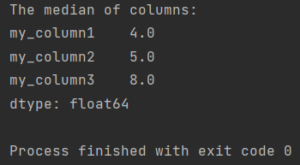Pandas How To Uncategorized How to calculate median in Pandas

# How to calculate median in Pandas

Here’s how to calculate median in Pandas.To calculate the median in Pandas use the median function.

## Median of a single column

```import pandas as pd

df1 = pd.DataFrame({"my_column1": ['9', '2', '3', '5'],
"my_column2": ['3', '7', '6', '4'],
"my_column3": ['4', '8', '8', '8']})

median = df1['my_column2'].median()

print(f'The median of my_column2 is equal to: {median}')

```

The result looks like this.

```Median of my_column2 equals: 5.0
```

To calculate the median of multiple columns in Pandas, use the median function.

## Median of many columns

```import pandas as pd

df1 = pd.DataFrame({"my_column1": ['9', '2', '3', '5'],
"my_column2": ['3', '7', '6', '4'],
"my_column3": ['4', '8', '8', '8']})

median = df1[['my_column2', 'my_column3']].median()

print(f'The median of my columns is equal to:\n{median}')

```

The result looks like this.

```The median of my columns is equal to:
my_column2    5.0
my_column3    8.0
dtype: float64
```

Remember to enter two pairs of square brackets. If you forget, you will get the following error:

```Traceback (most recent call last):
File "C:\Users\pandashowto\PycharmProjects\venv\lib\site-packages\pandas\core\indexes\base.py", line 3361, in get_loc
return self._engine.get_loc(casted_key)
File "pandas\_libs\index.pyx", line 76, in pandas._libs.index.IndexEngine.get_loc
File "pandas\_libs\index.pyx", line 108, in pandas._libs.index.IndexEngine.get_loc
File "pandas\_libs\hashtable_class_helper.pxi", line 5198, in pandas._libs.hashtable.PyObjectHashTable.get_item
File "pandas\_libs\hashtable_class_helper.pxi", line 5206, in pandas._libs.hashtable.PyObjectHashTable.get_item
KeyError: ('my_column2', 'my_column3')

The above exception was the direct cause of the following exception:

Traceback (most recent call last):
File "C:\Users\pandashowto\PycharmProjects\new.py", line 7, in
median = df1['my_column2', 'my_column3'].median()
File "C:\Users\pandashowto\PycharmProjects\venv\lib\site-packages\pandas\core\frame.py", line 3455, in __getitem__
indexer = self.columns.get_loc(key)
File "C:\Users\pandashowto\PycharmProjects\venv\lib\site-packages\pandas\core\indexes\base.py", line 3363, in get_loc
raise KeyError(key) from err
KeyError: ('my_column2', 'my_column3')

Process finished with exit code 1

```

## Median of all columns

You can also calculate the median of your entire dataframe. If you use the median method directly, you get the median of all columns.

```import pandas as pd

df1 = pd.DataFrame({"my_column1": ['9', '2', '3', '5'],
"my_column2": ['3', '7', '6', '4'],
"my_column3": ['4', '8', '8', '8']})

print(f'The median of columns:\n{df1.median()}')

```

The result looks like this.

```The median of columns:
my_column1    4.0
my_column2    5.0
my_column3    8.0
dtype: float64
```
Tags:

## How to replace part of string in PandasHow to replace part of string in Pandas

To replace part of a string in a Pandas DataFrame, you can use the str.replace() method with a regular expression. This allows you to replace substrings that match a specific

## How to remove duplicate rows based on multiple columnsHow to remove duplicate rows based on multiple columns

You can remove duplicate rows based on multiple columns in Pandas by using the drop_duplicates method. Here is an example of how you could remove duplicate rows based on multiple